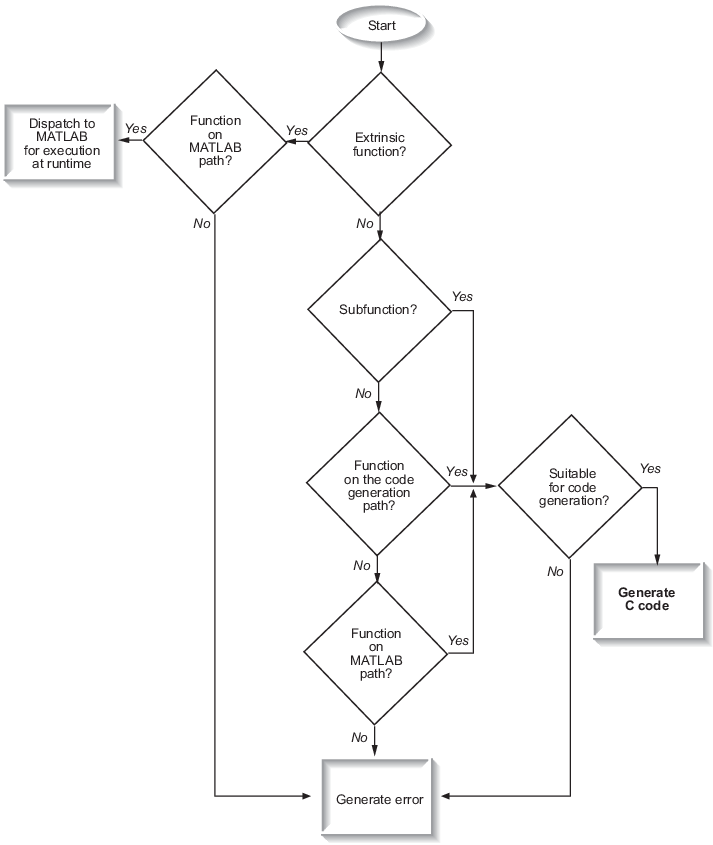## Resolution of Function Calls for Code Generation

From a MATLAB® function, you can call local functions, supported toolbox functions, and other MATLAB functions. MATLAB resolves function names for code generation as follows:### Key Points About Resolving Function Calls

The diagram illustrates key points about how MATLAB resolves function calls for code generation:

• Searches two paths, the code generation path and the MATLAB path

• Attempts to compile functions unless the code generator determines that it should not compile them or you explicitly declare them to be extrinsic.

If a MATLAB function is not supported for code generation, you can declare it to be extrinsic by using the construct `coder.extrinsic`, as described in Declaring MATLAB Functions as Extrinsic Functions. During simulation, the code generator produces code for the call to an extrinsic function, but does not generate the internal code for the function. Therefore, simulation can run only on platforms where MATLAB software is installed. During standalone code generation, the code generator attempts to determine whether the extrinsic function affects the output of the function in which it is called — for example by returning `mxArrays` to an output variable. If the output does not change, code generation proceeds, but the extrinsic function is excluded from the generated code. Otherwise, compilation errors occur.

The code generator detects calls to many common visualization functions, such as `plot`, `disp`, and `figure`. The software treats these functions like extrinsic functions but you do not have to declare them extrinsic using the `coder.extrinsic` function.

• Resolves file type based on precedence rules described in Resolution of File Types on Code Generation Path

### Compile Path Search Order

During code generation, function calls are resolved on two paths:

1. Code generation path

MATLAB searches this path first during code generation. The code generation path contains the toolbox functions supported for code generation.

2. MATLAB path

If the function is not on the code generation path, MATLAB searches this path.

MATLAB applies the same dispatcher rules when searching each path (see Function Precedence Order).

### When to Use the Code Generation Path

Use the code generation path to override a MATLAB function with a customized version. A file on the code generation path shadows a file of the same name on the MATLAB path.